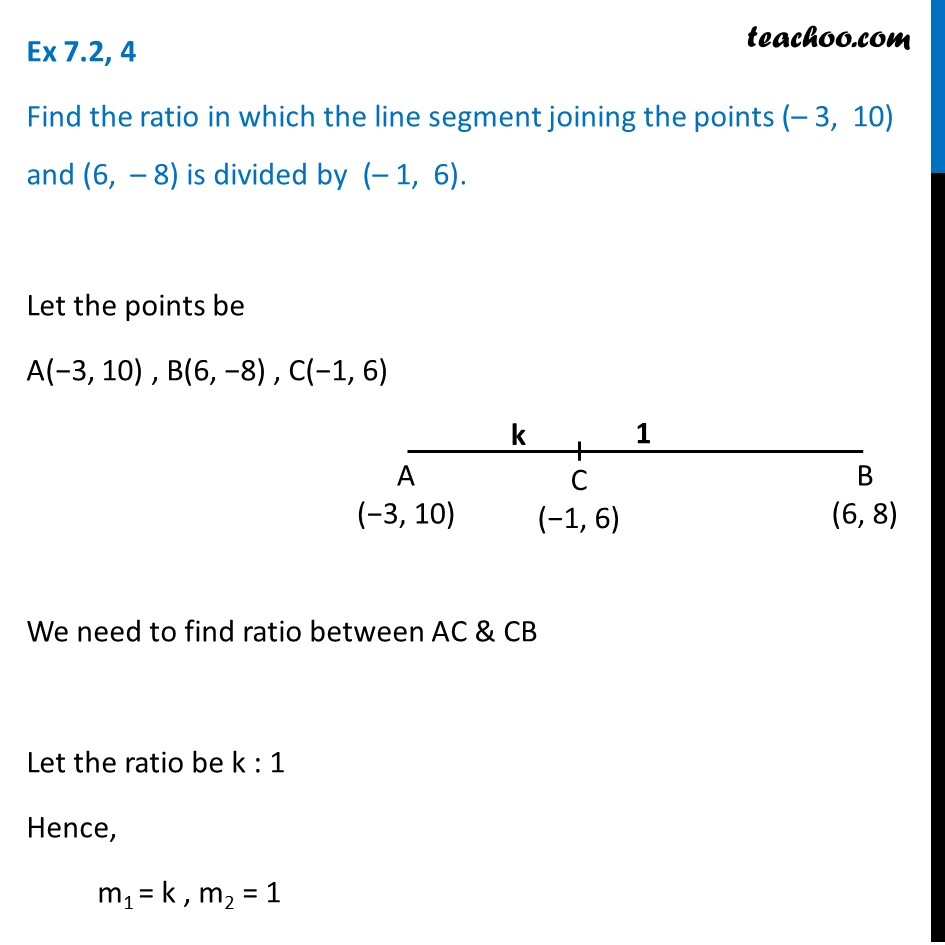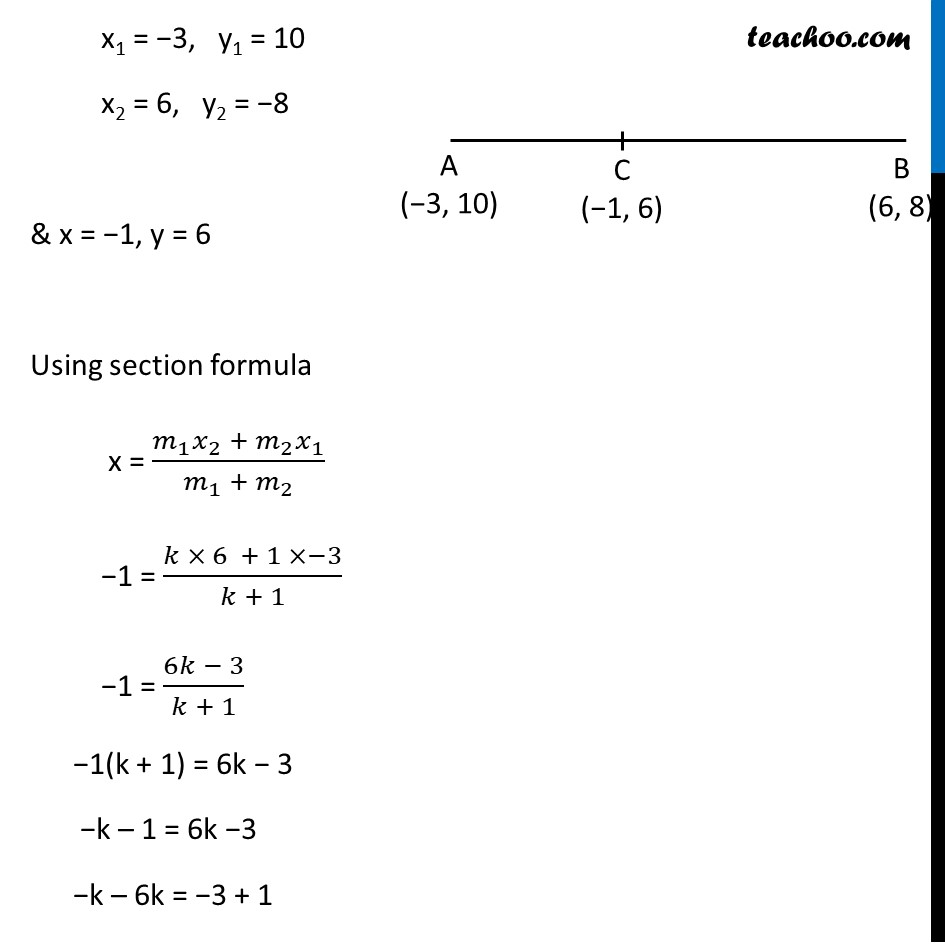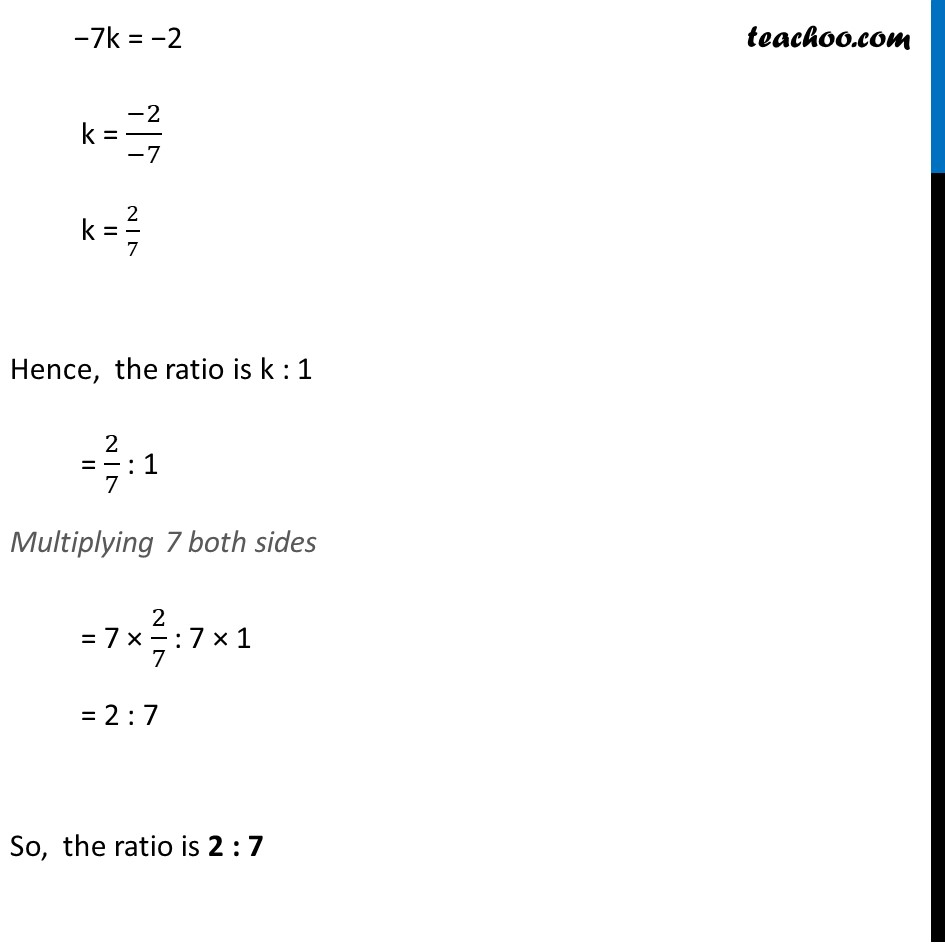Ex 7.2

Chapter 7 Class 10 Coordinate Geometry
Serial order wiseLearn in your speed, with individual attention - Teachoo Maths 1-on-1 Class

### Transcript

Ex 7.2, 4 Find the ratio in which the line segment joining the points (– 3, 10) and (6, – 8) is divided by (– 1, 6). Let the points be A(−3, 10) , B(6, −8) , C(−1, 6) We need to find ratio between AC & CB Let the ratio be k : 1 Hence, m1 = k , m2 = 1 x1 = −3, y1 = 10 x2 = 6, y2 = −8 & x = −1, y = 6 Using section formula x = (𝑚_1 𝑥_2 + 𝑚_2 𝑥_1)/(𝑚_1 + 𝑚_2 ) −1 = (𝑘 × 6 + 1 ×−3)/(𝑘 + 1) −1 = (6𝑘 − 3)/(𝑘 + 1) −1(k + 1) = 6k − 3 −k – 1 = 6k −3 −k – 6k = −3 + 1 −7k = −2 k = (−2)/(−7) k = 2/7 Hence, the ratio is k : 1 = 2/7 : 1 Multiplying 7 both sides = 7 × 2/7 : 7 × 1 = 2 : 7 So, the ratio is 2 : 7